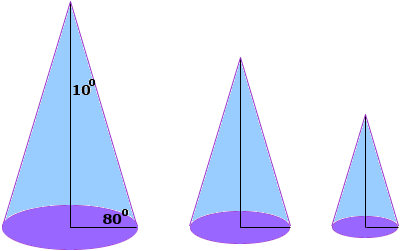I know the cone is 20 degrees at the top and 80 degrees at the bottom. What is the formula for finding the radius? Hi there. While you do indeed have enough information there to describe the shape of the cone, you don't have enough information to describe its size and therefore its radius. Using simple trigonometry you can see construct a right triangle with angles 80 degrees and 10 degrees (half the 20) where the bottom leg is the radius, the vertical leg is the height of the cone, and the hypotenuse is the sloped side.But the radius can only be expressed as a ratio using these quantities, for example, the radius is the height times tan(10). Stephen La Rocque.>